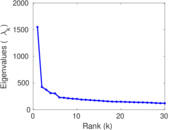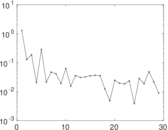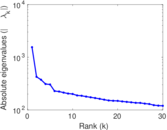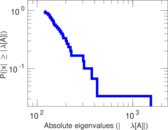# vi.sualize.us tag–item

This is the bipartite picture tagging network of vi.sualize.us. Left nodes represent tags and right nodes represents pictures. An edge connects a picture with an assigned tag.

 Code `Vti` Internal name `pics_ti` Name vi.sualize.us tag–item Data source http://vi.sualize.us/ AvailabilityDataset is available for download Consistency checkDataset passed all tests Category Feature network Node meaning Tag, picture Edge meaning Assignment Network formatBipartite, undirected Edge typeUnweighted, multiple edges

## Statistics

 Size n = 577,437 Left size n1 = 82,035 Right size n2 = 495,402 Volume m = 2,298,816 Unique edge count m̿ = 1,800,330 Wedge count s = 30,601,838,083 Claw count z = 1,985,799,614,059,094 Square count q = 2,199,351,548 4-Tour count T4 = 140,005,809,036 Maximum degree dmax = 405,429 Maximum left degree d1max = 405,429 Maximum right degree d2max = 790 Average degree d = 7.962 14 Average left degree d1 = 28.022 4 Average right degree d2 = 4.640 30 Fill p = 4.429 91 × 10−5 Average edge multiplicity m̃ = 1.276 89 Size of LCC N = 564,218 Diameter δ = 17 50-Percentile effective diameter δ0.5 = 3.468 93 90-Percentile effective diameter δ0.9 = 4.915 48 Median distance δM = 4 Mean distance δm = 3.887 09 Gini coefficient G = 0.764 600 Balanced inequality ratio P = 0.201 174 Left balanced inequality ratio P1 = 0.083 396 4 Right balanced inequality ratio P2 = 0.282 309 Tail power law exponent γt = 2.591 00 Degree assortativity ρ = −0.167 621 Degree assortativity p-value pρ = 0.000 00 Spectral norm α = 1,548.34 Algebraic connectivity a = 0.009 718 28 Spectral separation |λ1[A] / λ2[A]| = 3.644 49 Controllability C = 442,519 Relative controllability Cr = 0.766 350

## Plots

### Fruchterman–Reingold graph drawing### Degree distribution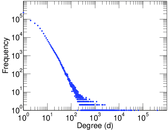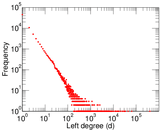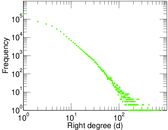### Cumulative degree distribution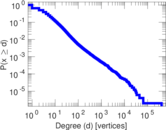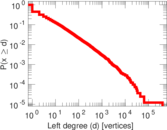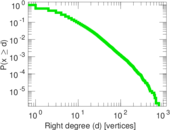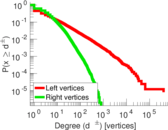### Lorenz curve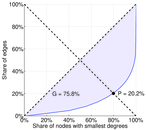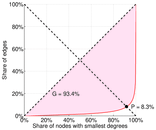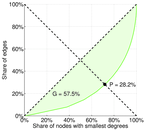### Spectral distribution of the adjacency matrix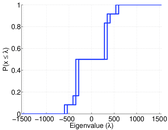### Spectral distribution of the normalized adjacency matrix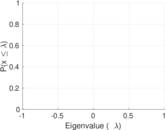### Spectral distribution of the Laplacian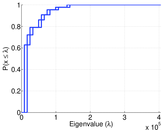### Spectral graph drawing based on the adjacency matrix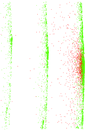### Spectral graph drawing based on the Laplacian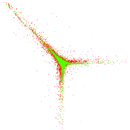### Spectral graph drawing based on the normalized adjacency matrix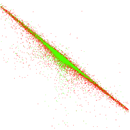### Degree assortativity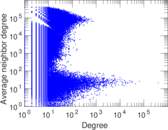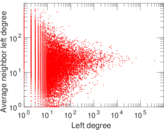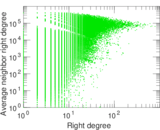### Zipf plot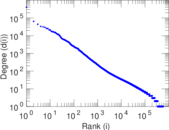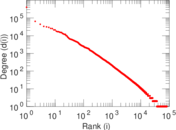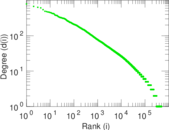### Hop distribution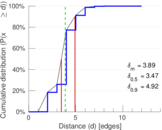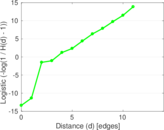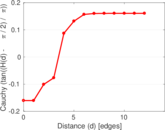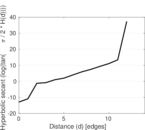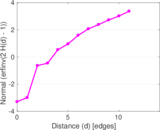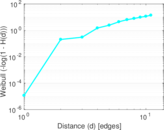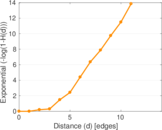### Edge weight/multiplicity distribution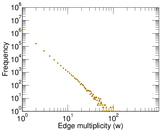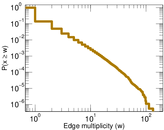### Matrix decompositions plots